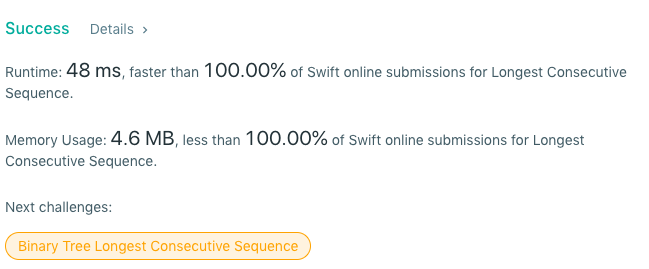This question is asked quite often in technical interview . The problem is this:

Write a function that takes in an unsorted array of integers and returns the longest range or longest consecutive sequence. For example:

`let array = [3,2,4,5,6,7,12,10,8,9,21,15,11,13,16,19,20,19]`

In this array there are 18 elements, however the longest consecutive goes from 2 to 13. You can simply eye ball the above array and see consecutive numbers from 2 all the way till 13. Then you have another range from 19 to 21 and another even smaller one that goes from 15 to 16.

The function should return the start and end values of the sequence.

I strongly urge to first try this problem out before checking out my version of this solution given below.

Alright, now let’s dive in to the solution. In my version of this solution, I am using only one Hash Map or Dictionary.The dictionary is of type `[Int : Bool]`. When we have used an integer successfully in creating a sequence the bool value should become true.

Here is the function signature:

Here I am initializing the dictionary and three variables to keep track of the longest sequence and in the for loop, I am giving value of false to all the entries of the dictionary.

This is the meat of the code. In this part, we are essentially first checking if the number we have landed on is already used in some other sequence by checking its value. If its true, we continue with the next iteration of the loop otherwise we continue. Then we are are setting `previousVal` and `nextValue` as current value `(n)` - 1 and current value + 1 respectively. By using two while loops, we are first looking for the previous value to the current number in the hash map. If the value for it exists or the key is available, the bool value is switched to true. If there is no value in the dictionary for the `previousVal` key the while loop ends and goes to the next loop where we check the next value in the loop. Again the same thing, adding 1 to the `nextVal` and checking its presence in the hash map.

Then at the end we are checking if the current two values of `nextValue` and `previousValue` has the highest amount of numbers between them by taking the difference and returning a string:

This is the output you get when you run this code:

Checking this algorithm in leetcode returns this result: